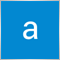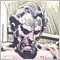# Return Standard Deviation of ATR?70

I want to return the standard deviation of the ATR over a given period.

Trying to do this, but it isn't working.

```double my_ATR = iATR(NULL, 0, my_ATR_period, 0);
double my_ATR_stddev = iStdDevOnArray(my_ATR, 0, my_ATR_period, 0, 0, 0);```

Any Ideas?3297

Any Ideas?

See if setting the number of elements to non-zero helps.

```double  iStdDevOnArray(
double       array[],          // array with data
int          total,            // number of elements
int          ma_period,        // MA averaging period
int          ma_shift,         // MA shift
int          ma_method,        // MA averaging method
int          shift             // shift
);```20233

Kila Akil: Trying to do this, but it isn't working.

Don't post code what won't even compile. Obviously the first argument must be an array. So get an array of values.70

whroeder1:

Don't post code what won't even compile. Obviously the first argument must be an array. So get an array of values.

Sorry.

How do I create an array that contains ATR values for the last x candles? (Array must update with each new bar).

i.e when a new bar is formed, the least-recent element of the array is dropped, all elements are then shifted back an index, and then a new ATR value is added as the final element.

example:

original array = a, b, c, d, e, f, g, h, i, j

**new bar forms**

updated array = b, c, d, e, f, g, h, i, j, k

**new bar forms**

updated array = c, d, e, f, g, h, i, j, k, l

etc.20233

No dropping, no shifting, no thinking. Just fill the array each time you need it.70

whroeder1:
No dropping, no shifting, no thinking. Just fill the array each time you need it.

Have I done this correctly?

```int ATRPeriod = 10;
double ATR_Array[];

double AverageTrueRange = iATR(NULL, 0, ATRPeriod, 0);
//Creating the ATR

int Array_Size = ArraySize(ATRPeriod);
//Defining the variable to set the ATR array size

ArrayResize(ATR_Array, size+1);
//Add an element to the array for a new value

ATR_Array[size] = AverageTrueRange;
//Add the ATR value to the new element of the Array

ArraySetAsSeries(ATR_Array, True);
//Reverse the Array

double ATR_StdDev = iStdDevOnArray(ATR_Array, ATRPeriod, ATRPeriod , 0, 0, 0);
//Calculate the standard deviation of the 10 most recent elements

ArraySetAsSeries(ATR_Array, False);
//Reset the order of the Array```3297

Kila Akil:

Have I done this correctly?

No, not really.

Try something like this:

```   const int ATR_PERIOD = 10;              // averaging period
const int STDEV_MA_PERIOD = 10;
const int NUM_VALUES_TO_FILL = 100;    // How many ATR values I want to put in my array
double ATR_Array[];

// Size the array
ArrayResize(ATR_Array, NUM_VALUES_TO_FILL);

// Loop over how many values to fill the array
for(int i = 0; i<NUM_VALUES_TO_FILL; i++)
{
// Fills each ATR value
ATR_Array[i] = iATR(NULL, 0, ATR_PERIOD, i);
Print(StringFormat("iATR for [%d] is [%.4f]", i, ATR_Array[i]));
}

// StDev is as StDev does.
double ATR_StdDev = iStdDevOnArray(ATR_Array, NUM_VALUES_TO_FILL, STDEV_MA_PERIOD, 0, 0, 0);
Print(StringFormat("StDev is [%.4f]", ATR_StdDev));```